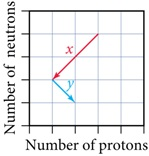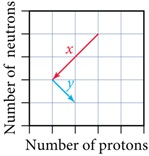# Problem: Consider the graphical representation of a series of decays shown here. The arrow labeled x and the arrow labeled y each correspond to what kind of decay?

###### FREE Expert Solution

We’re being asked to determine what kind of decay corresponds to the the arrows labeled x and y in the following graphRecall that in a nuclear reaction, the number of protons and neutrons is affected and the identity of the element changes

The different types of radioactive decay are:

• Alpha decay: forms an alpha particle (42α, atomic mass = 4, atomic number = 2)

• Beta decay: forms a beta particle (0–1β, atomic mass = 0, atomic number = –1). The beta particle appears in the product side.

• Gamma emission: forms a gamma particle (00γ, atomic mass = 0, atomic number = 0)

• Positron emission: forms a positron particle (01e, atomic mass = 0, atomic number = 1)

• Electron capture: the initial nuclide captures an electron
(0–1e, atomic mass = 0, atomic number = –1).
The electron appears in the reactant side.###### Problem Details

Consider the graphical representation of a series of decays shown here. The arrow labeled x and the arrow labeled y each correspond to what kind of decay?